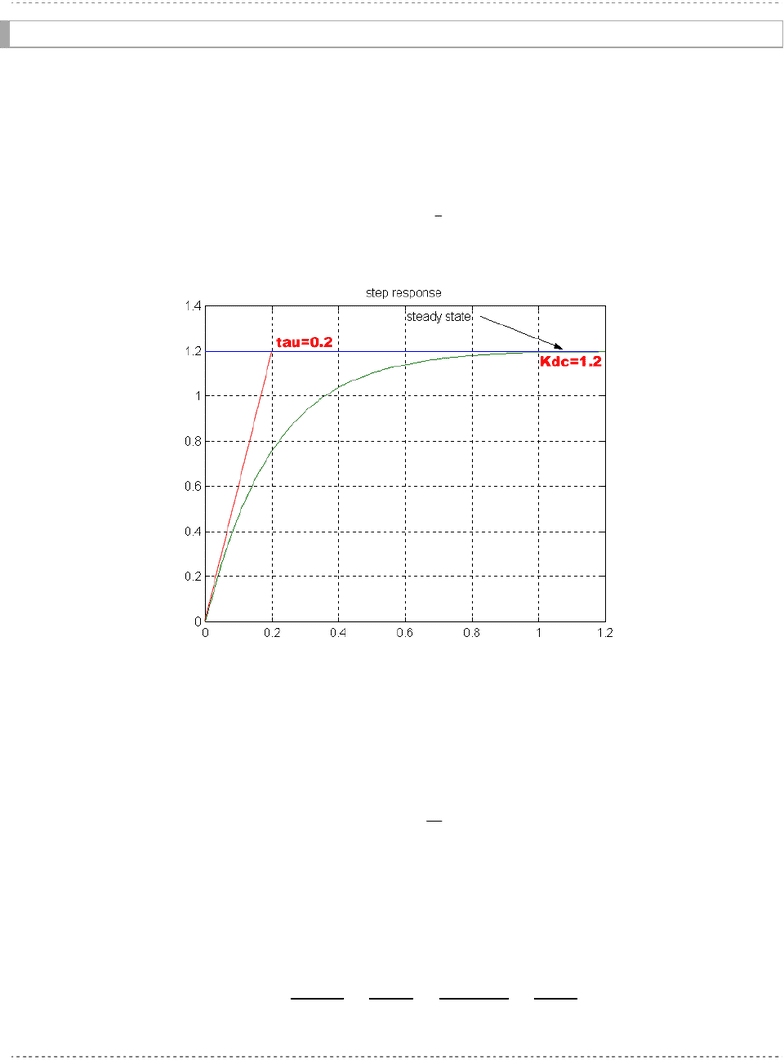# ELE 639 Lecture Notes - Lecture 6: Step Response, Damping Ratio, Domain Analysis

36 views6 pagesELE/BME639 Course Notes Winter 2011
Page 122
6 Time Domain Analysis: Dynamic Response Part 1
6.1 First Order Systems
A first order system is described by the transfer function in Equation 6-1:
1
)(
s
K
a
s
K
sG dc
Equation 6-1
G(s) has only one pole, and no zeros. Its unit step response can be derived as shown in Equation 6-2:
 
as
K
s
K
ass
K
sG
s
sY
21
)(
1
)(
a
K
s
K
K
a
K
as
K
K
as
s
2
0
1


)(11)(11)(
11
)(
teKte
a
K
ty
asa
K
sa
K
sY
t
dc
at
Equation 6-2
Note that:

00
)(1
dc
t
dc
K
dt
dy
te
K
dt
dy
Equation 6-3
If the unit step input is used, the process DC gain and time constant can be evaluated directly from the
graph, as shown in the following example.
Unlock document

This preview shows pages 1-2 of the document.
Unlock all 6 pages and 3 million more documents.ELE/BME639 Course Notes Winter 2011
Page 123
6.1.1 Example
Consider a plot of the response of a certain unknown process, shown in Figure 6-1. We would like to
derive a model for this unknown system, i.e. a transfer function that would give a response closest to that
of our system, let's call it (s). The response looks like an exponential rise with a non-zero slope at t=0,
and is therefore identified as the response of a first order process (system). As such, the response can be
described by the following equation:
)(11)( teKty t
dc
Figure 6-1 First Order System response
where the DC gain and the exponential Time Constant can be read off directly from the plot. Since
 1
and  
 ∙
, then  
 1.2. To read off the Time Constant, take a tangent to the exponential
rise and identify the cross-over of it with the steady state value of the output (here equal to 1.2). As
indicated in Figure 6-1, = 0.2 sec. Therefore:

)(112.1)(
)(112.1)(
5
2.0
tety
tety
t
t
The process model transfer function will be then:

  1
1.2
0.2  1 6
5
Unlock document

This preview shows pages 1-2 of the document.
Unlock all 6 pages and 3 million more documents.

## Document Summary

6 time domain analysis: dynamic response part 1. A first order system is described by the transfer function in equation 6-1: sg. G(s) has only one pole, and no zeros. Its unit step response can be derived as shown in equation 6-2: Note that: dy dt dy dt t e. If the unit step input is used, the process dc gain and time constant can be evaluated directly from the graph, as shown in the following example. Consider a plot of the response of a certain unknown process, shown in figure 6-1. We would like to derive a model for this unknown system, i. e. a transfer function that would give a response closest to that of our system, let"s call it (cid:1833)(cid:3040)(s). The response looks like an exponential rise with a non-zero slope at t=0, and is therefore identified as the response of a first order process (system). As such, the response can be described by the following equation: ty.

## Get access

\$10 USD/m
Billed \$120 USD annuallyHomework Help
Study Guides
Textbook Solutions
Class Notes
Textbook Notes
Booster Class